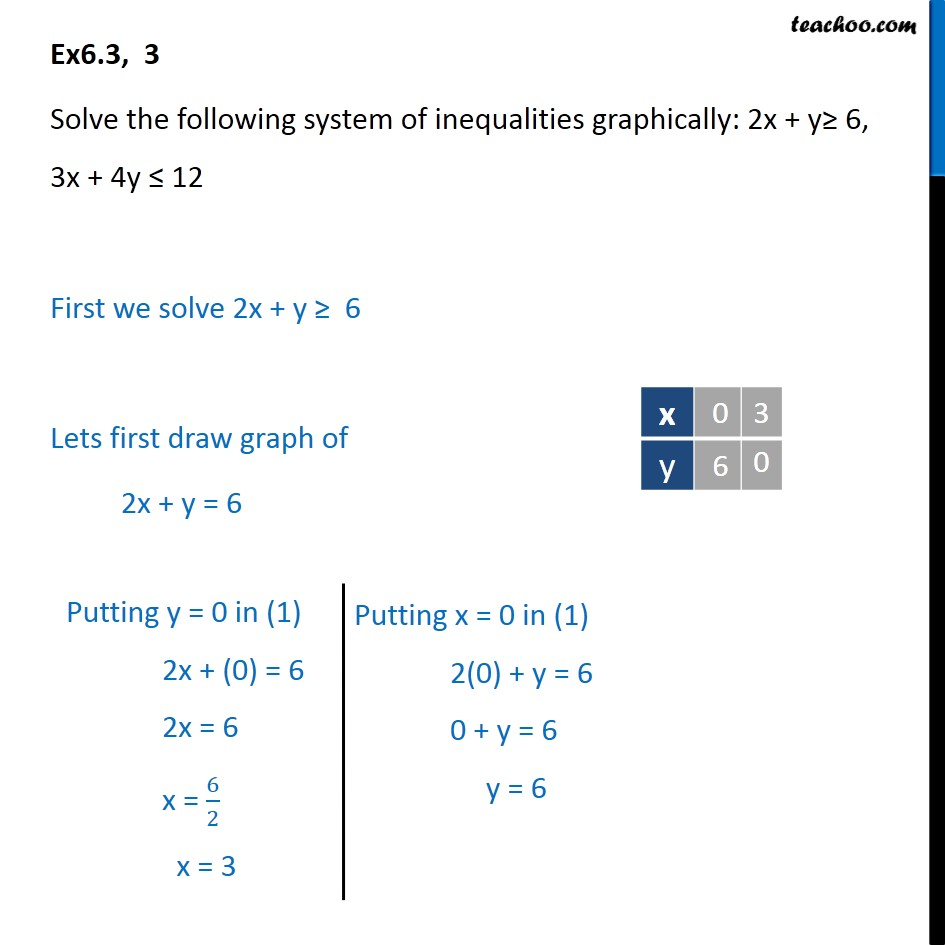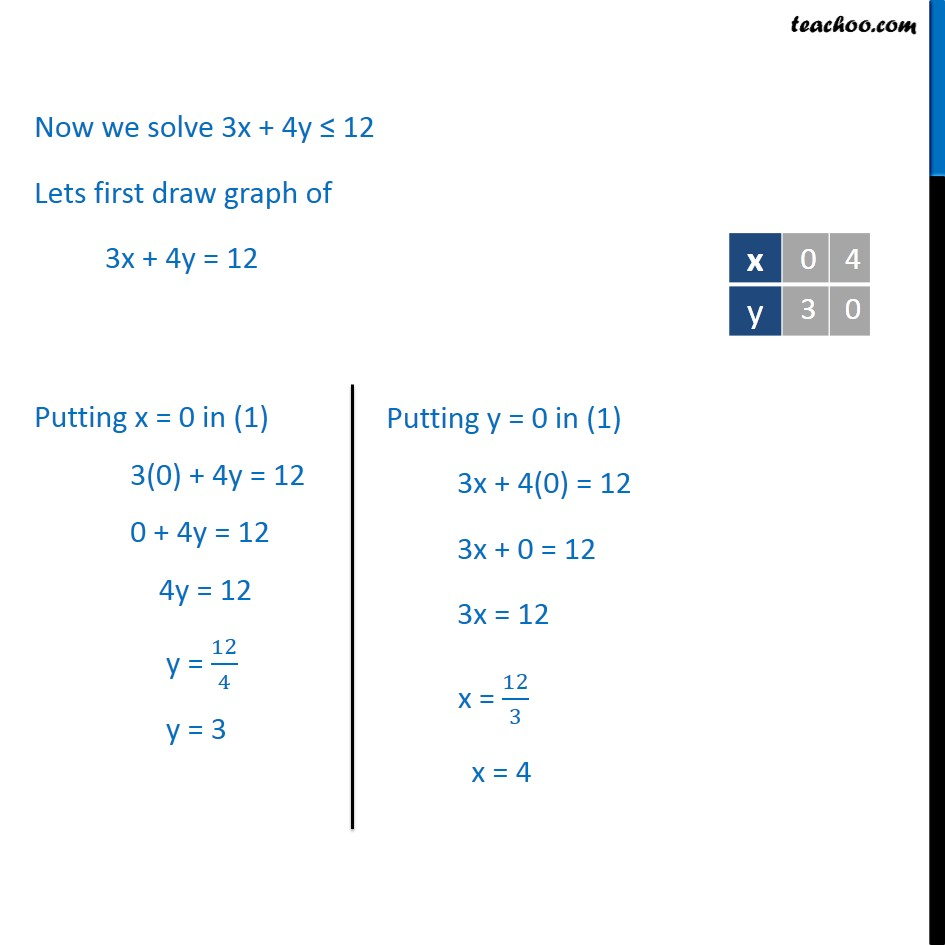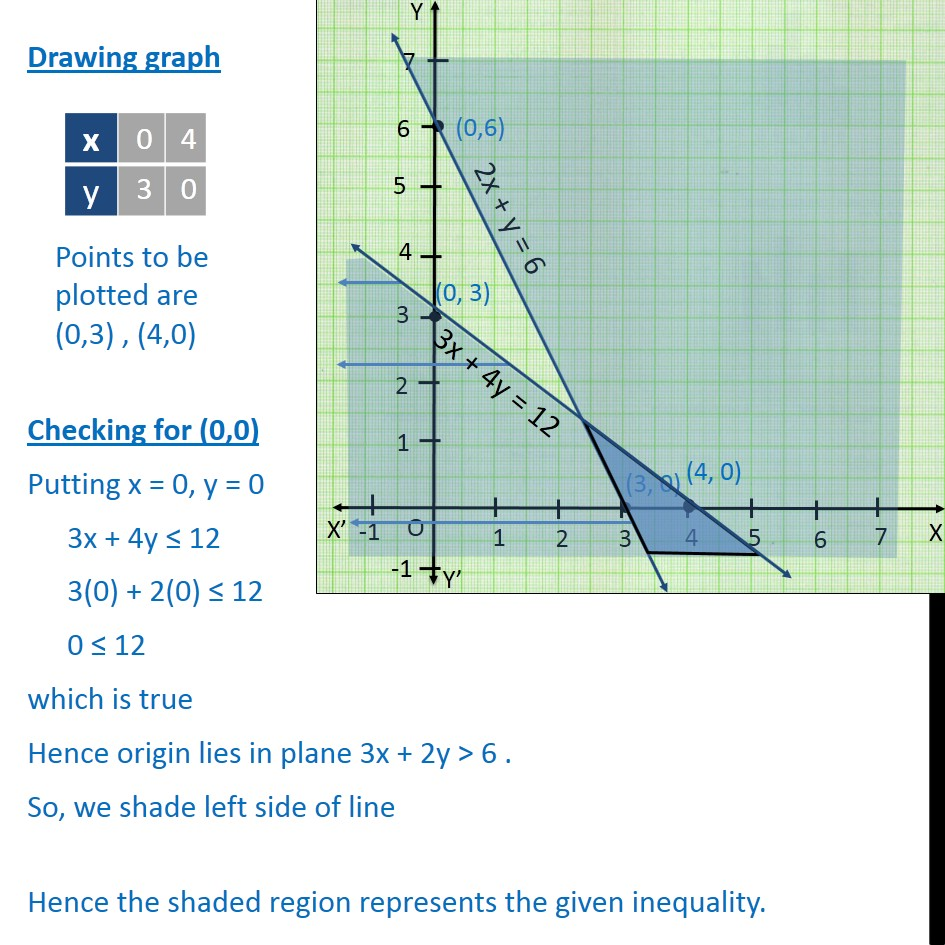Ex 6.3

Chapter 6 Class 11 Linear Inequalities (Term 2)
Serial order wise### Transcript

Ex6.3, 3 Solve the following system of inequalities graphically: 2x + y 6, 3x + 4y 12 First we solve 2x + y 6 Lets first draw graph of 2x + y = 6 Drawing graph Checking for (0,0) Putting x = 0, y = 0 2x + y 6 2(0) + (0) 6 0 6 which is false So, we shade right side of line Hence origin does not lie in plane 2x + y 6 Now we solve 3x + 4y 12 Lets first draw graph of 3x + 4y = 12 Drawing graph Checking for (0,0) Putting x = 0, y = 0 3x + 4y 12 3(0) + 2(0) 12 0 12 which is true Hence origin lies in plane 3x + 2y > 6 . So, we shade left side of line Hence the shaded region represents the given inequality.